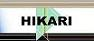| Home | Journals | Books | Paper submission | About us and our mission | News | Contact us |Site Search:

## Online Edition, Vol. 6, 2012, no. 49-52

W. F. H. Al-Shameri
Dynamical properties of the Henon mapping
Int. Journal of Math. Analysis, Vol. 6, 2012, no. 49-52, 2419-2430.

A. A. Hemeda
Homotopy perturbation method for solving partial differential equations of fractional order
Int. Journal of Math. Analysis, Vol. 6, 2012, no. 49-52, 2431-2448.

Shaochun Ji, Gang Li
Existence and controllability results for fractional integrodifferential equations with impulsive and nonlocal conditions
Int. Journal of Math. Analysis, Vol. 6, 2012, no. 49-52, 2449-2458.

R. K. Muthumalai
Note on Newton interpolation formula
Int. Journal of Math. Analysis, Vol. 6, 2012, no. 49-52, 2459-2465.

M. I. Ismailov, A. N. Jabrailova
On vector-valued analogy of Riesz-Fisher, Hardy and Littlewood theorems
Int. Journal of Math. Analysis, Vol. 6, 2012, no. 49-52, 2467-2472.

Seong-Hoon Cho
Fixed point theorems for generalized contractive mappings on cone metric spaces
Int. Journal of Math. Analysis, Vol. 6, 2012, no. 49-52, 2473-2481.

Some more results on facto graphs
Int. Journal of Math. Analysis, Vol. 6, 2012, no. 49-52, 2483-2492.

Md. Iftekhar Salam, HoonJae Lee
Algebraic analysis of shrinking generator
Int. Journal of Math. Analysis, Vol. 6, 2012, no. 49-52, 2493-2499.

A. M. Mathai
Generalized Kratzel integral and associated statistical densities
Int. Journal of Math. Analysis, Vol. 6, 2012, no. 49-52, 2501-2510.

Kumar Ashok, Taneja H. C., Chitkara Ashok K., Kumar Vikas
Classification of census using information theoretic measure based ID3 algorithm
Int. Journal of Math. Analysis, Vol. 6, 2012, no. 49-52, 2511-2518.

A. Ouahab, A. Mbarki, J. Masude
On the Ishikawa process for (a,b)-mean nonexpansive mappings fixed point by approximated sequences
Int. Journal of Math. Analysis, Vol. 6, 2012, no. 49-52, 2519-2526.

A. Kumari, B. L. Raina, H. B. Singh
Fast convergent modified Newton method
Int. Journal of Math. Analysis, Vol. 6, 2012, no. 49-52, 2527-2537.

B. Yousefi, Gh. R. Moghimi
On the Banach spaces of formal power series
Int. Journal of Math. Analysis, Vol. 6, 2012, no. 49-52, 2539-2544.

C. S. Ryoo
On the q-Euler polynomials with weak weight \alpha associated with the p-adic q-integrals on Z_p
Int. Journal of Math. Analysis, Vol. 6, 2012, no. 49-52, 2545-2553.

Dae Won Yoon, Ji Soon Jun
Non-degenerate quadric surfaces in Euclidean 3-space
Int. Journal of Math. Analysis, Vol. 6, 2012, no. 49-52, 2555-2562.

Ing-Jer Lin, Shu-Huei Yang
Some existence and convergence theorems of best proximity point for new nonlinear cyclic maps
Int. Journal of Math. Analysis, Vol. 6, 2012, no. 49-52, 2563-2578.

J. Cheshmavar
A survey on periodic wavelets
Int. Journal of Math. Analysis, Vol. 6, 2012, no. 49-52, 2579-2583.

Liangwei Wang
Critical exponent on asymptotic behavior of rescaled solutions for porous medium equation with absorptions
Int. Journal of Math. Analysis, Vol. 6, 2012, no. 49-52, 2585-2590.

R. Srikanth, S. Subburam
The solution-free Diophantine equation y^2=a_nx^n+a_{n - 1}x^{n - 1} + ... + a_0
Int. Journal of Math. Analysis, Vol. 6, 2012, no. 49-52, 2591-2596.

B. Chacko
Cech closure operators on a fixed set
Int. Journal of Math. Analysis, Vol. 6, 2012, no. 49-52, 2597-2603.